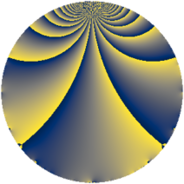# Properties

 Label 925.2.bLevel $925$ Weight $2$ Character orbit 925.b Rep. character $\chi_{925}(149,\cdot)$ Character field $\Q$ Dimension $54$ Newform subspaces $9$ Sturm bound $190$ Trace bound $11$

# Related objects

## Defining parameters

 Level: $$N$$ $$=$$ $$925 = 5^{2} \cdot 37$$ Weight: $$k$$ $$=$$ $$2$$ Character orbit: $$[\chi]$$ $$=$$ 925.b (of order $$2$$ and degree $$1$$) Character conductor: $$\operatorname{cond}(\chi)$$ $$=$$ $$5$$ Character field: $$\Q$$ Newform subspaces: $$9$$ Sturm bound: $$190$$ Trace bound: $$11$$ Distinguishing $$T_p$$: $$2$$, $$3$$, $$7$$

## Dimensions

The following table gives the dimensions of various subspaces of $$M_{2}(925, [\chi])$$.

Total New Old
Modular forms 102 54 48
Cusp forms 90 54 36
Eisenstein series 12 0 12

## Trace form

 $$54 q - 46 q^{4} - 12 q^{6} - 38 q^{9} + O(q^{10})$$ $$54 q - 46 q^{4} - 12 q^{6} - 38 q^{9} - 4 q^{11} + 4 q^{14} + 30 q^{16} - 28 q^{21} + 36 q^{24} + 4 q^{26} + 12 q^{29} + 4 q^{31} + 40 q^{34} + 6 q^{36} - 12 q^{39} - 8 q^{41} + 32 q^{44} - 64 q^{46} - 74 q^{49} - 8 q^{51} - 40 q^{54} + 12 q^{56} + 4 q^{59} - 48 q^{61} + 14 q^{64} + 104 q^{66} + 12 q^{69} - 52 q^{71} - 10 q^{74} + 60 q^{76} - 28 q^{79} - 10 q^{81} + 44 q^{84} + 20 q^{86} + 24 q^{89} - 112 q^{94} - 116 q^{96} + 12 q^{99} + O(q^{100})$$

## Decomposition of $$S_{2}^{\mathrm{new}}(925, [\chi])$$ into newform subspaces

Label Dim $A$ Field CM Traces $q$-expansion
$a_{2}$ $a_{3}$ $a_{5}$ $a_{7}$
925.2.b.a $2$ $7.386$ $$\Q(\sqrt{-1})$$ None $$0$$ $$0$$ $$0$$ $$0$$ $$q+2iq^{2}+iq^{3}-2q^{4}-2q^{6}+5iq^{7}+\cdots$$
925.2.b.b $2$ $7.386$ $$\Q(\sqrt{-1})$$ None $$0$$ $$0$$ $$0$$ $$0$$ $$q+2iq^{2}-3iq^{3}-2q^{4}+6q^{6}+iq^{7}+\cdots$$
925.2.b.c $2$ $7.386$ $$\Q(\sqrt{-1})$$ None $$0$$ $$0$$ $$0$$ $$0$$ $$q+iq^{2}+2iq^{3}+q^{4}-2q^{6}-2iq^{7}+\cdots$$
925.2.b.d $2$ $7.386$ $$\Q(\sqrt{-1})$$ None $$0$$ $$0$$ $$0$$ $$0$$ $$q-iq^{3}+2q^{4}+3iq^{7}+2q^{9}-5q^{11}+\cdots$$
925.2.b.e $2$ $7.386$ $$\Q(\sqrt{-1})$$ None $$0$$ $$0$$ $$0$$ $$0$$ $$q-iq^{3}+2q^{4}-iq^{7}+2q^{9}+3q^{11}+\cdots$$
925.2.b.f $10$ $7.386$ $$\mathbb{Q}[x]/(x^{10} + \cdots)$$ None $$0$$ $$0$$ $$0$$ $$0$$ $$q-\beta _{1}q^{2}+(\beta _{2}+\beta _{8})q^{3}+(-2+\beta _{3}+\cdots)q^{4}+\cdots$$
925.2.b.g $10$ $7.386$ 10.0.$$\cdots$$.1 None $$0$$ $$0$$ $$0$$ $$0$$ $$q-\beta _{8}q^{2}-\beta _{7}q^{3}+(-2+\beta _{1}-\beta _{6}+\cdots)q^{4}+\cdots$$
925.2.b.h $10$ $7.386$ 10.0.$$\cdots$$.1 None $$0$$ $$0$$ $$0$$ $$0$$ $$q+\beta _{1}q^{2}+(\beta _{1}-\beta _{3})q^{3}+\beta _{2}q^{4}+(-1+\cdots)q^{6}+\cdots$$
925.2.b.i $14$ $7.386$ $$\mathbb{Q}[x]/(x^{14} + \cdots)$$ None $$0$$ $$0$$ $$0$$ $$0$$ $$q+\beta _{1}q^{2}+\beta _{9}q^{3}+(-1+\beta _{2})q^{4}+(1+\cdots)q^{6}+\cdots$$

## Decomposition of $$S_{2}^{\mathrm{old}}(925, [\chi])$$ into lower level spaces

$$S_{2}^{\mathrm{old}}(925, [\chi]) \cong$$ $$S_{2}^{\mathrm{new}}(185, [\chi])$$$$^{\oplus 2}$$